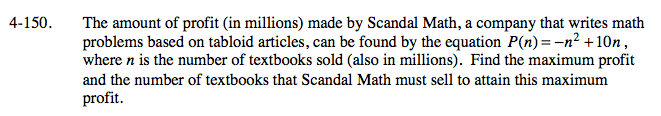### Home > A2C > Chapter 4 > Lesson 4.3.2 > Problem4-150

4-150.To find the maximum, you need to find the vertex of the parabola. Write the equation in graphing form so you can find the vertex.

It is easier to complete the square without the −n2. Start by multiplying both sides by −1 so −P(n) = n2 − 10n. Now complete the square.

Do not forget to multiply again by −1 after you complete the square.

The vertex is your maximum y-value.